Alternating Current(AC) and AC Steady State Analysis
Complex Numbers and Phasors

 Outline: Sinusoidal Sources Review of Complex Numbers AC Steady State Analysis Definition of a Phasor AC Analysis using Phasors

Sinusoidal Sources

This chapter covers analysis of circuits when the source is sinusoidal.
The analysis techniques are exactly the same as those used when the source
was DC. What makes AC analysis more difficult is the mathematics, as will
be seen in the next section. Fortunately there are mathematical tools and
short cuts, such as using Phasors.

Review of Sinusoidal Sources

A sinusoidal source has the following attributes:
• An amplitude
• A frequency
• A Phase Angle

The following expession represents an AC source.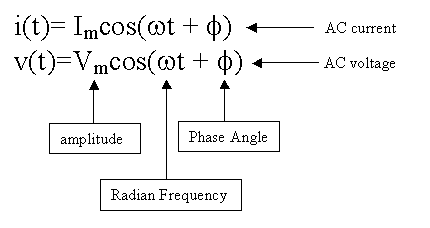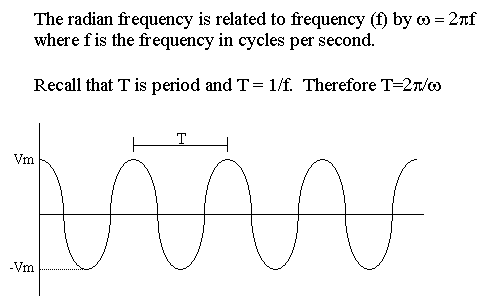Here are some examples of AC sources: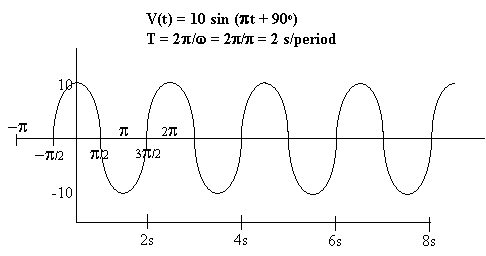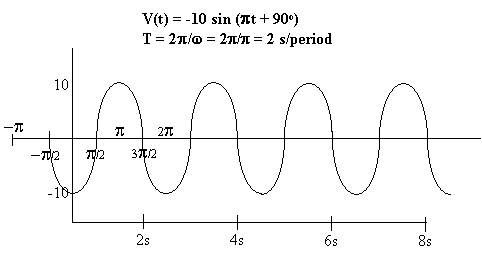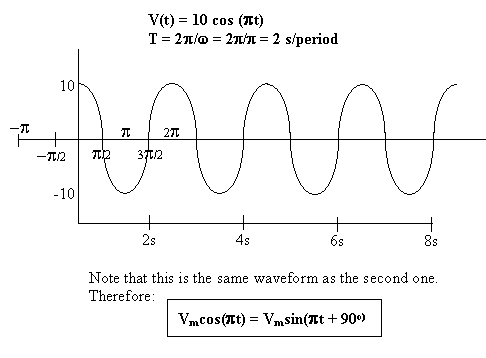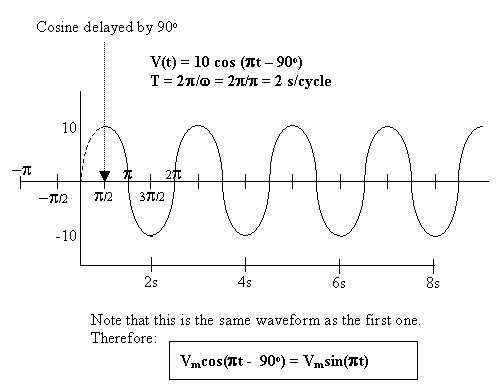Top of Page

Review of Complex Numbers

In order to perform AC analysis, one must understand how to
work with complex numbers.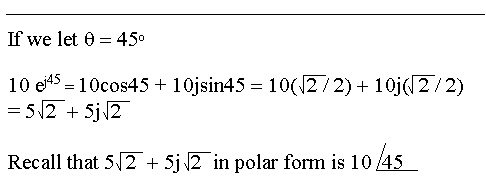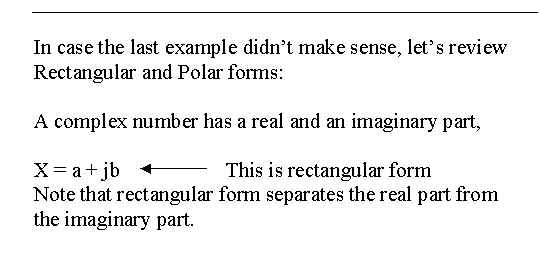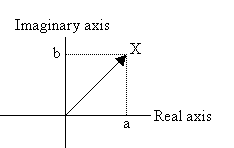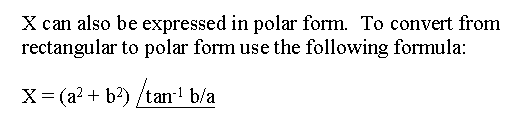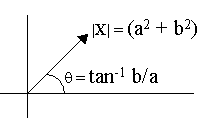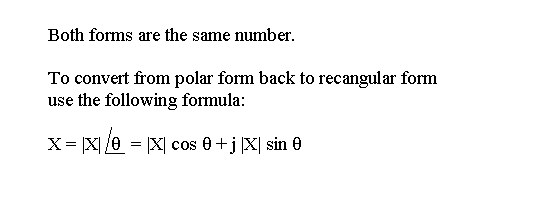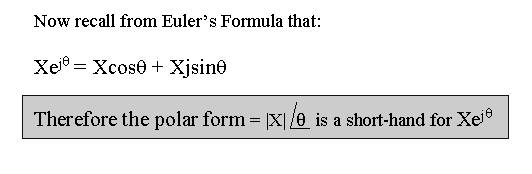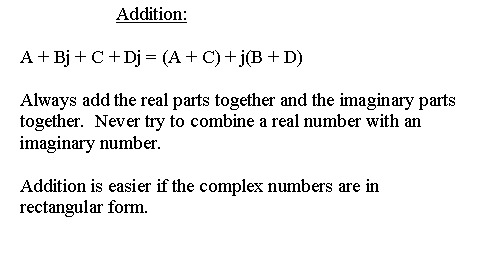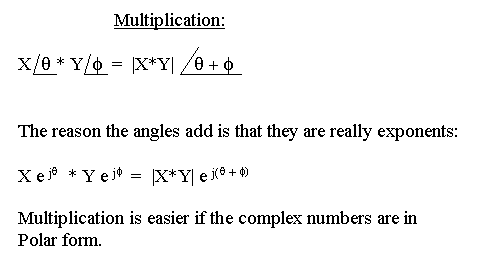Show why is j*j = -1?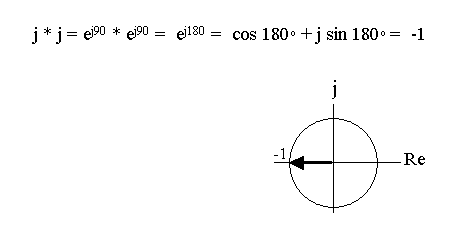Example: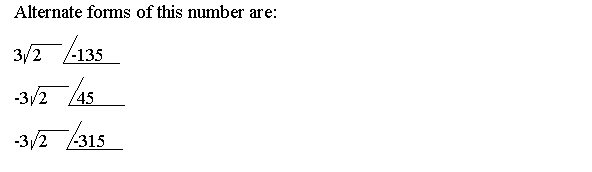Top of Page

We are now ready to analyze a simple circuit with an AC Source.
Do not panic because after we introduce phasors this problem will
be significantly easier.

 This AC Circuit is in Steady State. This means that all of the AC voltages and currents are constant (not flat like DC but unchanging). Note there are many detailed steps, but if you can follow this problem then you will be able to grasp what a phasor is in the next section.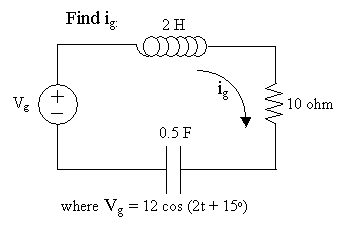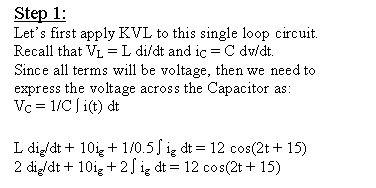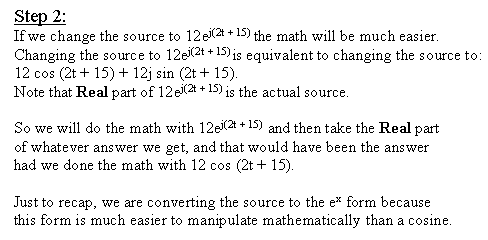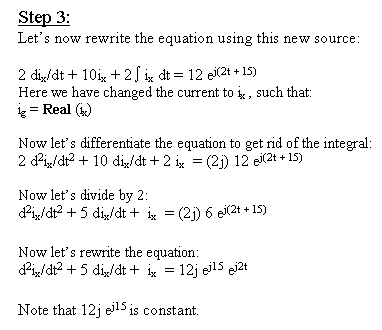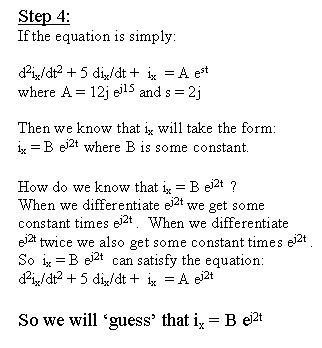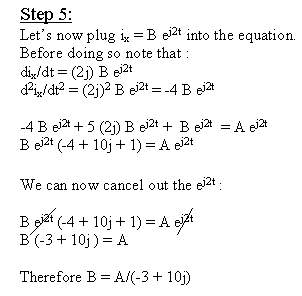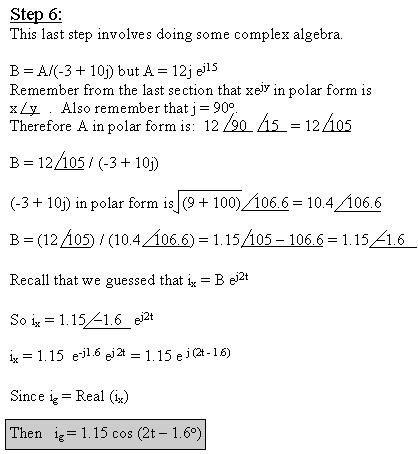Note that in this example the ej2t cancelled out of the math. Therefore to determine B we did not need the ej2t. The radian frequency (the '2' in this case) is only needed when differentiating the KVL equation. (see step 3) In summary, the radian frequency determines the coefficients of the equation but after step 3 the radian frequency is simply in the way.

As we will see in the next section, the introduction of the phasor
helps simplify the math by eliminating the radian frequency from the math.

Top of Page

Definition of a Phasor

A phasor represents the magnitude and phase ONLY of a voltage or current.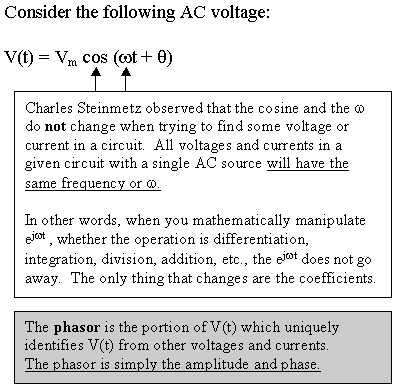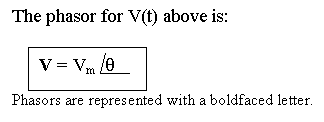In this table we will convert each voltage or current into its phasor form:
 V = 30 cos(10t - 45o) The phasor for V is: V = 30/-45 or V = -30/135 I = -5 cos(5t + 30o) The phasor for I is: I = -5/30 or I = 5/-150 I = -10 sin(3t - 30o) Here we will convert from sine to cosine: As seen in section one, we subtract 90o to convert from sine to cosine. I = -10 cos(3t -30o - 90o) = -10 cos(3t - 120o) Also recall from math that cos(wt) = -cos(wt +/- 180o) So I = 10 cos(3t - 120o + 180o) = 10 cos(3t + 60o) The phasors for I are: I = -10/-120 or I = 10/60 V = 30 sin(10t - 180o) Again we convert from sine to cosine: V = 30 cos(10t - 180o - 90o) = 30 cos(10t - 270o) V = 30 cos(10t - 270o + 360o) = 30 cos(10t + 90o) The phasors for V are: V = 30/-270 or V = 30/90 Note that there are many ways of writing one phasor. As long as each phasor value represents the same vector when graphed, then all are equivalent.

Summary

Phasors are a very compact form of writing amplitude and phase for a voltage or current.

Top of Page

AC Analysis using Phasors

Let's begin analysis using phasors by considering single element circuits.
We will consider a resistor circuit, a capacitor circuit, and an inductor circuit.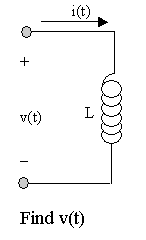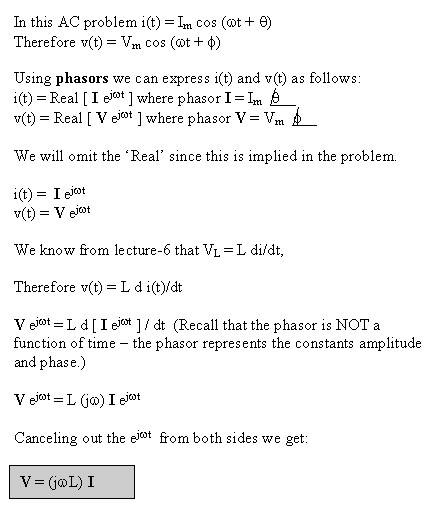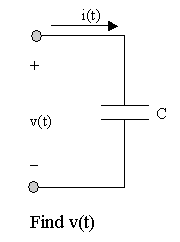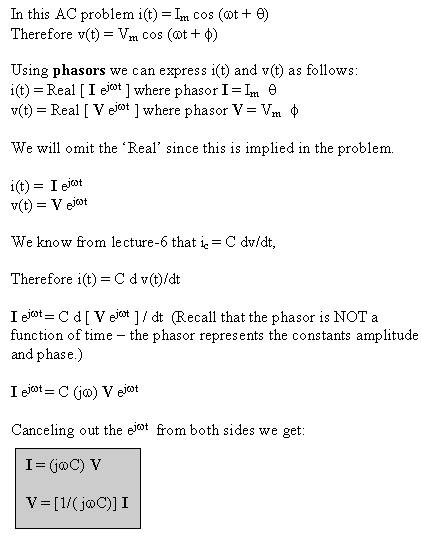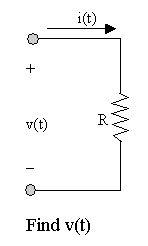One can see that Ohm's Law, V=IR, holds true for phasors. The above Voltage-Current relationships for the Inductor, the Capacitor, and the resistor will significantly simplify AC analysis.

Summary

We can now apply Ohm's Law to Inductors, Capacitors, and Resistors using Phasors.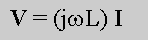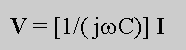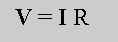## Study Problems

After clicking on the following link enter 9-1 for the problem and 1 for the step:
Study Problem 9-1

After clicking on the following link enter 9-2 for the problem and 1 for the step:
Study Problem 9-2

After clicking on the following link enter 9-3 for the problem and 1 for the step:
Study Problem 9-3

After clicking on the following link enter 9-4 for the problem and 1 for the step:
Study Problem 9-4
problem in the section 'AC Steady State Analysis'
only this time phasors are used.

After clicking on the following link enter 9-5 for the problem and 1 for the step:
Study Problem 9-5

Top of Page

Back To Index